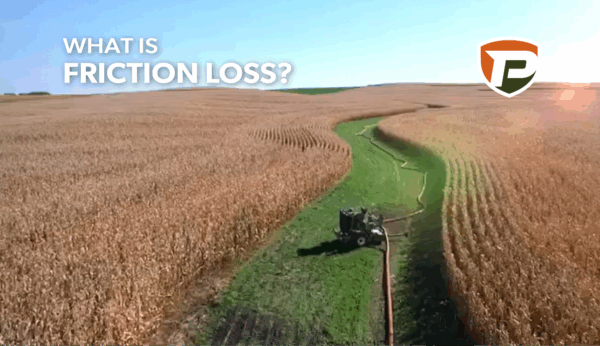February 10, 2021

# What Is Friction Loss?

Many different elements need to be factored into planning a hose system. One of the essential aspects of the planning process is understanding the pumps' capabilities and the hose system's limitations. The limitation that we are referring to is a fluid transfer process called friction loss. Knowing what friction loss factors allows operators to gauge the best approach to overcoming this limitation.

## So what is friction loss exactly?

Essentially, friction loss is a form of energy consumption by the interaction between the flowing liquid and the material that it is moving through.

More specifically, when liquid flows through a hose line, friction occurs between the fluid and the hose's internal wall. This friction causes fluid stresses (depending on its viscosity) and turbulence. Having turbulence in the flow slows down the fluid and results in a drop in PSI (pressure loss) at the end of the hose.

Since hoses consume flow and pressure, the hose's diameter presents an opportunity to help dampen some of the challenges posed by friction loss. Larger diameter hoses allow a higher flow rate, and at a fixed rate, more distance can be covered using the same number of pumps than with a small diameter hose. However, while applicators want to hit those targeted flow rates, the higher the amount of GPM going through the hose, the greater the turbulence and friction loss.

Elevation also plays a part in the amount of pressure loss in the hose. Pushing liquid uphill will increase pressure loss while pushing liquid downhill will result in a gain in pressure. This factor should be taken into consideration when setting up hoses. The amount of pressure loss in the hose amounts to around 0.43 PSI for every foot in elevation.

Even though that might not seem like a lot, it can tally up very quickly. For example, if you are pumping the liquid up a 100-foot high hill, the elevation alone will result in a loss of 43 PSI at the other end of the hose.

Fiction loss is why we utilize a system of pumps to make sure we can continue to create pressure and flow in the hoses from point A to point B. But in order for applicators to do this properly, it takes planning to know what the capabilities of your pumps are and how much hose you will need to get the job done.

## How to Calculate Pipe/Hose Friction Loss

There are a few different ways to calculate the loss of pressure due to friction. The formula we point our customers to is called the Hazen-Williams Formula. For imperial units, this equation is:

`hf = 0.002083 x l x (100 / c)1.852 x (q1.852 / d4.8655)`

Where:

hf= head loss in free of water
l= length of hose
c= Hazen-Williams Formula pipe material roughness constant (160 for layflat hoses)
q = volume flow (gal/min)
d= inside hydraulic diameter (inches)

For example, let's see what the friction loss would be for a 660 ft length of 7-inch diameter layflat hose with a targeted flow rate of 2,500 gallons per minute.

hf = 0.002083 x l x (100 / c)1.852 x (q1.852 / d4.8655)
hf = 0.002083 x 660 x (100 / 160)1.852 x (2,5001.852 / 74.8655)
hf = 0.002083 x 660 x 0.419 x (1,963,291.99 / 12,936.73)
hf = 0.002083 x 660 x 0.419 x (1,963,291.99 / 12,936.73)
hf = 0.002083 x 660 x 0.419 x 151.76
hf = 87.42 ft of liquid loss at the head of the hose

To have more rapid calculations, we've put together a chart that acts as a quick reference for estimating the amount of friction loss for a certain length of hose (660 ft) given the hose diameter and flow rate (in gallons per minute).

If we track down the variables used in our example equation on the chart, you can see that at the intersection of the 7-inch hose (y-axis) and the 2,500 GPM flow rate (x-axis) that we have a head (pressure) loss of 87 feet. This closely matches the results found in our formula example above. The chart also allows you to identify the loss of PSI in the hose line and the velocity of the fluid. When we look at the 87-foot head loss scenario, we can also see that the head loss PSI would be 38 PSI, and the loss in velocity would be 21 feet per second.

## With these calculations, you can figure out how many pumps you will need to overcome friction loss.

When you're in a rush and cannot do the calculations on your own, sites like The Engineering Toolbox can help calculate friction loss. For more in-depth coverage and explanations of friction loss, check out Puck's Pump School to find a class that's convenient for you and your crew.

For technical support regarding the topic covered in this article or any other question, the Puck team is here for you with trained specialists at the ready. You can contact Puck's technical support by calling 712.655.9200 or submit a request online